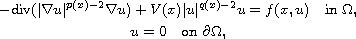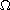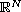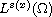Electron. J. Diff. Equ., Vol. 2016 (2016), No. 197, pp. 1-19.

### Existence and multiplicity of solutions for a Dirichlet problem involving perturbed p(x)-Laplacian operator Aboubacar Abdou, Aboubacar Marcos

Abstract:
In this article we study the existence of solutions for the Dirichlet problemwhereis a smooth bounded domain in, V is a given function in a generalized Lebesgue spaceand f(x,u) is a Caratheodory function which satisfies some growth condition. Using variational arguments based on "Fountain theorem" and "Dual Fountain theorem", we shall prove under appropriate conditions on the above nonhomogeneous quasilinear problem the existence of two sequences of weak solutions for this problem.

Submitted December 18, 2015. Published July 24, 2016.
Math Subject Classifications: 35B38, 35J20, 35J60, 35J66, 58E05.
Key Words: p(x)-Laplacian operator; generalized Lebesgue-Sobolev spaces; critical point; Fountain theorem; dual Fountain theorem.

Show me the PDF file (298 KB), TEX file for this article.Aboubacar Abdou Institut de Mathématiques et de Sciences Physiques Université d'Abomey Calavi, 01 BP: 613 Porto-Novo, Bénin email: aboubacar.abdou@imsp-uac.org, abdou.aboubacar@ymail.com Aboubacar Marcos Institut de Mathématiques et de Sciences Physiques Université d'Abomey Calavi, 01 BP: 613 Porto-Novo, Bénin email: abmarcos@imsp-uac.org, abmarcos@yahoo.fr